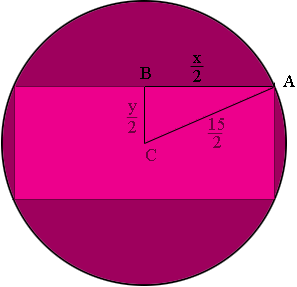SEARCH HOMEMath Central Quandaries & QueriesQuestion from Alexea, a student: A rectangle is inscribed in a circle of diameter 15in. Express the perimeter as a function of the width x.Hi Alexea,

Let the height of the rectangle by $y$ then the perimeter is $2x + 2y.$In my diagram $ABC$ is a right triangle. What does Pythagoras tell you? Solve for $y$ in terms of $x.$ Substitute into the perimeter expression.

PennyMath Central is supported by the University of Regina and The Pacific Institute for the Mathematical Sciences.